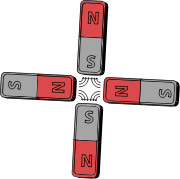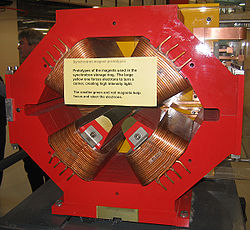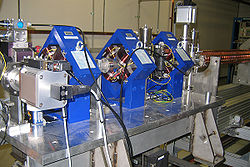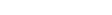xEncyclopediaQuadrupole magnets consist of groups of four magnet
Magnet
A magnet is a material or object that produces a magnetic field. This magnetic field is invisible but is responsible for the most notable property of a magnet: a force that pulls on other ferromagnetic materials, such as iron, and attracts or repels other magnets.A permanent magnet is an object...

s laid out so that in the multipole expansion
Multipole expansion
A multipole expansion is a mathematical series representing a function that depends on angles — usually the two angles on a sphere. These series are useful because they can often be truncated, meaning that only the first few terms need to be retained for a good approximation to the original...

of the field the dipole terms cancel and where the lowest significant terms in the field equations are quadrupole
A quadrupole or quadrapole is one of a sequence of configurations of—for example—electric charge or current, or gravitational mass that can exist in ideal form, but it is usually just part of a multipole expansion of a more complex structure reflecting various orders of complexity.-Mathematical...

. Quadrupole magnets are useful as they create a magnetic field
Magnetic field
A magnetic field is a mathematical description of the magnetic influence of electric currents and magnetic materials. The magnetic field at any given point is specified by both a direction and a magnitude ; as such it is a vector field.Technically, a magnetic field is a pseudo vector;...

whose magnitude grows rapidly
Linear function
In mathematics, the term linear function can refer to either of two different but related concepts:* a first-degree polynomial function of one variable;* a map between two vector spaces that preserves vector addition and scalar multiplication....

In classical geometry, a radius of a circle or sphere is any line segment from its center to its perimeter. By extension, the radius of a circle or sphere is the length of any such segment, which is half the diameter. If the object does not have an obvious center, the term may refer to its...

distance from its longitudinal axis. This is used in particle beam
Particle beam
A particle beam is a stream of charged or neutral particles which may be directed by magnets and focused by electrostatic lenses, although they may also be self-focusing ....

focusing.

The simplest magnetic quadrupole is two identical bar magnets parallel to each other such that the north pole of one is next to the south of the other and vice versa. Such a configuration would have no dipole moment, and its field will decrease at large distances faster than that of a dipole. A stronger version with very little external field involves using a k=3 halbach cylinder.

In some designs of quadrupoles using electromagnet
Electromagnet
An electromagnet is a type of magnet in which the magnetic field is produced by the flow of electric current. The magnetic field disappears when the current is turned off...

s there are four steel
Steel
Steel is an alloy that consists mostly of iron and has a carbon content between 0.2% and 2.1% by weight, depending on the grade. Carbon is the most common alloying material for iron, but various other alloying elements are used, such as manganese, chromium, vanadium, and tungsten...

pole tips: two opposing magnetic north poles and two opposing magnetic south poles. The steel is magnetized by a large electric current
Electric current
Electric current is a flow of electric charge through a medium.This charge is typically carried by moving electrons in a conductor such as wire...

that flows in the coils of tubing wrapped around the poles. Another design is a Helmholtz coil
Helmholtz coil
A Helmholtz coil is a device for producing a region of nearly uniform magnetic field. It is named in honor of the German physicist Hermann von Helmholtz.- Description :A Helmholtz pair consists of two identical circular magnetic...

layout but with the current in one of the coils reversed.

At the speeds reached in high energy particle accelerator
Particle accelerator
A particle accelerator is a device that uses electromagnetic fields to propel charged particles to high speeds and to contain them in well-defined beams. An ordinary CRT television set is a simple form of accelerator. There are two basic types: electrostatic and oscillating field accelerators.In...

s, magnetic deflection is more powerful than electrostatic, and use of the magnetic term of the Lorentz force
Lorentz force
In physics, the Lorentz force is the force on a point charge due to electromagnetic fields. It is given by the following equation in terms of the electric and magnetic fields:...

:is enabled with various magnets that make up 'the lattice' required to bend, steer and focus
Strong focusing
In accelerator physics strong focusing or alternating-gradient focusing is the principle that the net effect on a particle beam of charged particles passing through alternating field gradients is to make the beam converge...

a charged particle beam. The quadrupoles in the lattice are of two types: 'F quadrupoles' (which are horizontally focusing but vertically defocusing) and 'D quadrupoles' (which are vertically focusing but horizontally defocusing). This situation is due to the laws of electromagnetism
Electromagnetism
Electromagnetism is one of the four fundamental interactions in nature. The other three are the strong interaction, the weak interaction and gravitation...

(the Maxwell equations) which show that it is impossible for a quadrupole to focus in both planes at the same time.

If an F quadrupole and a D quadrupole are placed immediately next to each other, their fields completely cancel out (in accordance with Earnshaw's theorem
Earnshaw's theorem
Earnshaw's theorem states that a collection of point charges cannot be maintained in a stable stationary equilibrium configuration solely by the electrostatic interaction of the charges. This was first proven by British mathematician Samuel Earnshaw in 1842. It is usually referenced to magnetic...

). But if there is a space between them (and the length of this has been correctly chosen), the overall effect is focusing in both horizontal and vertical planes. A lattice can then be built up enabling the transport of the beam over long distances—for example round an entire ring. A common lattice is a FODO lattice consisting of a basis of a focusing quadrupole, 'nothing' (often a bending magnet), a defocusing quadrupole and another length of 'nothing'.

• Charged particle beam
Charged particle beam
A charged particle beam is a spatially localized group of electrically charged particles that have approximately the same velocity . The kinetic energies of the particles are typically measured in keV or MeV, much larger than the energies of particles at ambient temperature...

• Dipole magnet
Dipole magnet
A dipole magnet, in particle accelerators, is a magnet constructed to create a homogeneous magnetic field over some distance. Particle motion in that field will be circular in a plane perpendicular to the field and collinear to the direction of particle motion and free in the direction orthogonal...

• Electron optics
Electron optics
Electron optics deals with the focusing and deflection of electrons using magnetic and/or electrostatic fields.- Electron properties :Electrons are charged particles . The electron also has an associated spin of + 1/2...

• Halbach cylinder
• Sextupole magnet
Sextupole magnet
Sextupole magnets consist of groups of six magnets set out in an arrangement of alternating north and south magnetic poles arranged around an axis. They are used in particle beam control in particle accelerators....